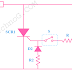## SCR(Silicon Controlled Rectifier) Applications

The main application of SCR is Switching. Actually, the switching property of the SCR(Silicon Controlled Rectifier) is used for any application.

The important Applications of SCR are,

(1) Silicon Controlled Rectifiers are used as Switch in electrical as well as electronic circuits.

(2) Silicon Controlled Rectifiers are used in DC Circuit Breakers.

(3) There is a huge application of Silicon Controlled Rectifiers in Inverter Circuits.

(4) Silicon Controlled Rectifiers are used in Electronic Chopper circuit.

(5) In controlled Rectifier Circuits SCRs are used.

(6) SCRs are used in Soft Starter.

(7) Silicon Controlled Rectifiers are used to control the speed of Electrical Motors.

(8) SCRs are used in AC Voltage Stabilizer circuits.

(9) SCRs are also used in Battery Charger circuits.

(10) SCRs are used to control electrical power.

(11) SCRs are used in Pulse Width Modulation Circuits.

(12) Silicon Controlled Rectifiers are used in Light Dimming circuits.

(13) Silicon Controlled Rectifiers are used in Solid State Relays.

(14) SCRs are used as Static contactor.

(15) SCRs are used for High voltage protection purpose.

(16) SCRs are used in power regulator devices.

Some of the Applications of SCR are explained here,

### Use of SCR as Switch:

The circuit diagram for SCR as a switch is given below,

Suppose the load draws above 100 amperes current from the source in the above diagram. So in this case, if we connect a switch between the supply and load to control the load current then there will be huge sparking. But if we use an SCR between them, the sparking can be minimized. And we control that SCR with a low voltage and current power supply. Basically, we use SCR as an electronically controlled switch.

As you see in the above diagram, during the positive half cycle SCR1 will be forward bias. Now if we close the switch then the Gate current will be applied to the Gate of the SCR through the diode D1 and Resistor R1. Hence the SCR1 starts to conduct current and the current also flows through the load.

Again during the Negative half cycle, SCR2 will be forward bias. Now if we close the switch then gate current will be applied to the Gate of the SCR through the diode D2 and Resistor R2. Hence the SCR starts conducting and current will flow through the load.

So you may understand we can control 100 amperes current by the controlling the few milliamperes Gate current of the SCR and there will be no sparking occurred.

### Application of SCR in Over Voltage Protection:

The switching property of the SCR can be used for over voltage protection. Here the circuit is given below. The overvoltage protection circuit is also called Crowbar Circuit.

When an overvoltage occurs then the Zener Diode starts conducting and current will flow through the Zener diode and the resistor R1. As a current flows through the resistor so a voltage will appear across the resistor which causes to flow the Gate current of the SCR. When SCR starts conducting, the voltage will be dropped by the SCR and the Load will be protected from the overvoltage. Here a snubber circuit is used for the protection of the SCR. It is a very economical circuit for voltage protection.

### SCR application in Chopper Circuits:

Using the Chopper Circuit we can step up or step down the DC voltage. We already discussed How Electronic Chopper Steps Up or Steps down the DC voltage? you can read that article. Here only the basic circuit diagram of Step Up Chopper and Step Down Chopper are given below.

The Chopper Circuit which Steps Up the DC voltage is called Step Up Chopper.

### Use of SCR for speed control of Motor:

We can use SCR to control the speed of the electrical motors. Here a circuit diagram for speed control of DC series Motor using SCR is given below,

As you see in the above diagram, here an SCR is connected in series with the load. A DIAC is connected to the Gate terminal of the SCR. When the AC supply is given to the Circuit, Capacitor starts charging through the field and armature winding. When the capacitor is fully charged it causes to flow a current through the DIAC and the gate terminal of the SCR. So the SCR gets triggered and it starts conducting, therefore, the motor will be run.

By changing the value of the variable resistance we can increase or decrease the charging time of the Capacitor. By changing the charging time of the capacitor we can control the speed of the Motor.

### Rectifier Circuit Using SCR:

Here a single-phase half-wave rectifier circuit using SCR is given below. An SCR is connected in series with the load. A Diode and a variable resistance are connected with the gate terminal of the SCR.
During the negative half cycle, the SCR will be reverse bias so it does not conduct.
During the positive half cycle, the SCR will be forward bias. Now by changing the value of the resistance, gate current can be given to the SCR. Hence the SCR gets triggered and it starts conducting. As a result, we will get the DC voltage across the load.

By changing the resistance we can change the firing angle of the SCR to control the average output voltage.

(1) The switching operation using SCR is very safe because here we can control a large amount of current or voltage by controlling a small amount of current.

(2) SCR is a static device it has no moving part, that is why it is noiseless.

(3) The SCR is a very highly efficient device.

(4) The switching speed of the SCR is very high.

(5) Smaller in size.

(6) Power Loss is very Low.

Thank you for visiting the website. keep visiting for more updates.
[Explained] Applications and Advantages of SCRReviewed by Author on March 15, 2019 Rating: 5